Courses

# Strength Of Materials 4

## 10 Questions MCQ Test Mock Test Series for Civil Engineering (CE) GATE 2020 | Strength Of Materials 4

Description
This mock test of Strength Of Materials 4 for Civil Engineering (CE) helps you for every Civil Engineering (CE) entrance exam. This contains 10 Multiple Choice Questions for Civil Engineering (CE) Strength Of Materials 4 (mcq) to study with solutions a complete question bank. The solved questions answers in this Strength Of Materials 4 quiz give you a good mix of easy questions and tough questions. Civil Engineering (CE) students definitely take this Strength Of Materials 4 exercise for a better result in the exam. You can find other Strength Of Materials 4 extra questions, long questions & short questions for Civil Engineering (CE) on EduRev as well by searching above.
QUESTION: 1

### In a closed coiled helical spring, the maximum shear stress occurs on the

Solution:

The major stresses in a helical spring are of two types, shear stress due to torsion and direct shear due to applied load. It is observed that for both tensile load as well as compressive load on the spring, maximum shear stress always occurs at the inner side of the spring. Hence, failure of the spring, in the form of crake, is always initiated from the inner radius of the spring.

QUESTION: 2

### A rigid bar AB of length ‘L’ is supported by a hinge and two springs of stiffness ‘K’ as shown in figure. The buckling load Pcr for the bar will be-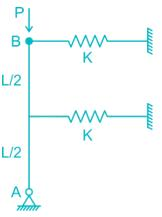Solution: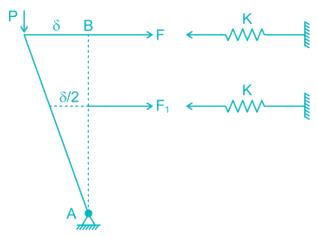F = Kδ and F1 = K × δ/2

Pδ = F × L + F1 × L/2
⇒ P × δ = Kδ × L + K × δ/2 × L/2
⇒ P = 5KL/4

QUESTION: 3

### If 10 coils of spring cut out from a spring of 25 coils, then the stiffness of new spring will be

Solution: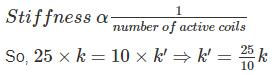⇒k′ = 2.5 k

QUESTION: 4

A bar of length L is supported by springs of stiffness Coefficient kA, kB, and a Torsional spring of torsional stiffness coefficient kT as shown in the figure below. The critical load is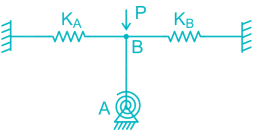Solution:

MA = 0

P x Δ = (KAΔ +KBΔ) L + KT θ

P = (KA+KB)L+KT/L

*Answer can only contain numeric values
QUESTION: 5

A column has a rectangular section of 10 mm x 20 mm & effective length of 1 m. The value of buckling stress (in MPa) is, given that E=200 GPa

Solution: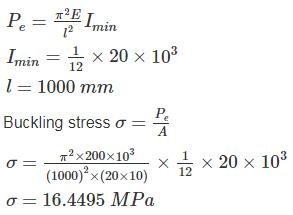*Answer can only contain numeric values
QUESTION: 6

A weight of 40 N falls from a height of 200 mm on a rigid massless bar supported by two identical springs as shown in the figure. If the maximum deflection is 20 mm then the stiffness of spring (in N/mm) is ………..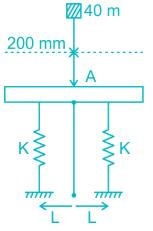Solution:

mg(h+x) = 2 × 1/2 kx2

40 ×(200+x) = kx2

40 ×(200+x) = k ×(20)2

k = 22 N/mm

QUESTION: 7

The euler’s crippling load for a column whose one end is fixed and other free is 60 kN. If one end is made fixed and other hinged, then the crippling load will

Solution:

End Condition - Effective length (Le)
Both end hinged - L
One end fixed other free - 2L
Both end fixed - 1/2
One end fixed and other hinged - L/√2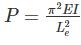Calculation: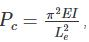where Le = effective length of column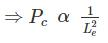Effective length for 1st case = 2L, effective length for 2nd case = L/√2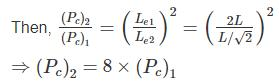QUESTION: 8

An a aluminium column of square cross-section (10 mm × 10 mm) and length 300 mm has both ends pinned. This is replaced by a circular cross-section (of diameter 10 mm) column of the same length and made of the same material with the same end conditions. The ratio of critical stresses for these two columns corresponding to Euler’s critical load, (σcritical)square : (σcirtical)circular’ is

Solution: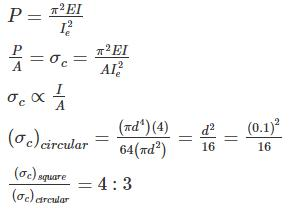*Answer can only contain numeric values
QUESTION: 9

A column has a rectangular cross section of 40 cm × 15 cm and of flexural rigidity 225 Nm2. If the column gets buckle under the axial load of 1110 N. Calculate the slenderness ratio of the column.

Solution:

Given A = 0.15 × 0.4 m2

Flexural rigidity: EI =225 Nm2

Pe = 1110 N

We know
I = 1/12 × 0.153 × 0.4 = 1.125 × 10−4 m4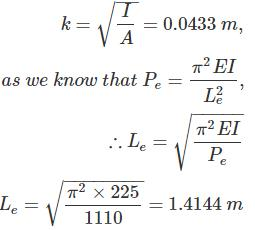Slenderness Ratio = S = Le/k = 32.665

*Answer can only contain numeric values
QUESTION: 10

Two beams are connected by a linear spring as shown in the following figure. For a load P as shown in the figure, the percentage of the applied load P carried by the spring is _______________.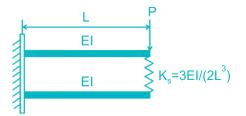Solution: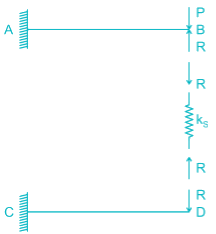Δspring = Δ− ΔD

Compression of spring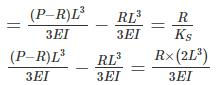(P – R) – R = 2R

P = 4R

R = P/4

% force carried by spring = 25%